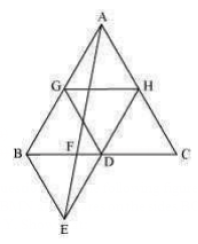Guru

# In Fig.9.33, ABC and BDE are two equilateral triangles such that D is the mid-point of BC. If AE intersects BC at F, show that:(i) ar (BDE) =1/4 ar (ABC) Q.5 (1)

• 0

How i solve the question of class 9th ncert math of Areas of Parallelograms and Triangles chapter of exercise 9.4 of question no 5(1). I think it is very important question of class 9th give me the tricky way for solving this question In Fig.9.33, ABC and BDE are two equilateral triangles such that D is the mid-point of BC. If AE intersects BC at F, show that:(i) ar (BDE) =1/4 ar (ABC)

Share

1. Assume that G and H are the mid-points of the sides AB and AC respectively.

Join the mid-points with line-segment GH. Here, GH is parallel to third side.

, BC will be half of the length of BC by mid-point theorem.∴ GH =1/2 BC and GH || BD

∴ GH = BD = DC and GH || BD (Since, D is the mid-point of BC)

Similarly,

GD = HC = HA

HD = AG = BG

, ΔABC is divided into 4 equal equilateral triangles ΔBGD, ΔAGH, ΔDHC and ΔGHD

We can say that,

ΔBGD = ¼ ΔABC

Considering, ΔBDG and ΔBDE

BD = BD (Common base)

Since both triangles are equilateral triangle, we can say that,

BG = BE

DG = DE

, ΔBDG ΔBDE [By SSS congruency]

, area (ΔBDG) = area (ΔBDE)

ar (ΔBDE) = ¼ ar (ΔABC)

Hence proved

• 0